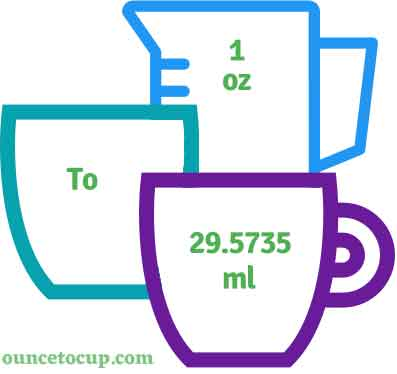# 1/4 Ounce to Milliliter (1/4 oz to ml conversion)

Are you cooking your favorite dish? The detailed chart in the recipe includes the calculation of 1/4 ounces to ml conversion? Do not worry; check this conversion tool to find how many 1/4 ounces equal to ml in a minute. This 1/4 oz to ml converter gives an exact measurement for any recipe you prepare.

1/4 is equal to 0.25. Then, 0.25 oz is equal to 7.3934 ml

Ounce Value:

Ounces

Milliliter Value:

ml

1/4 Ounce = 7.3934 Milliliter
(1/4 oz = 7.3934 ml)

Try our auto 1/4 ounce to milliliter calculator (Without Convert Button), Just change the first field value and you got final value.## How many ml is 1/4 oz?

We know that the volume value of 1/4 oz is equal to 7.3934 ml. If you want to convert 1/4 fluid oz to an equal number of ml, just multiply the volume value by 29.5735. Hence, 1/4 Ounce is equal to 7.3934 ml.

The Answer is: 1/4 US Fluid Ounces = 7.3934 US ml

1/4 oz = 7.3934 ml

Many of them try to search or find an answer for what is 1/4 ounces in ml? So, we’ll start with 1/4 fl to oz ml conversion to know how big is 1/4 oz.

## How To Calculate 1/4 fluid oz to ml?

To calculate 1/4 fluid ounces to an equal number of milliliter, simply follow the steps below.

Fluid Ounces to Milliliters formula is:

Milliliter = Fluid Ounce * 29.5735

Assume that we are finding out how many ml were found in 1/4 fl oz of water, multiply by 29.5735 to get the result.

Applying to Formula: ml = 1/4 oz * 29.5735 = 7.3934 ml.

## How To Convert 1/4 oz to ml?

• To convert 1/4 fluid ounces to ml,
• Simply multiply the 1/4 fluid ounces by 29.5735.
• Applying to the formula, ml = 1/4 ounces * 29.5735 [(1/4)x29.5735].
• Hence, 1/4 ounces is equal to 7.3934 ml.

## Some quick table references for fluid ounce to milliliter conversions:

Ounce [oz] Milliliter [ml]
1 oz29.5735 ml
2 oz59.147 ml
3 oz88.7205 ml
4 oz118.294 ml
5 oz147.8675 ml
6 oz177.441 ml
7 oz207.0145 ml
8 oz236.588 ml
9 oz266.1615 ml
10 oz295.735 ml
11 oz325.3085 ml
12 oz354.882 ml

## Reverse Calculation: How many ounces are in 1/4 ml?

• To convert 1/4 ml to oz,
• Simply divide the 1/4 ml by 29.5735.
• Then, applying the formula, ounce = 1/4 ml / 29.5735 [(1/4)/29.5735 = 0.008453506].
• Hence, 1/4 ml is equal to 0.008453506 ounce.

### Related Converter:

Formula: Ounce to ml

ml = ounce / 29.5735

Applying to Formula,

ml = (1/4)/29.5735 = 7.3934

1/4 oz = 7.3934 ml